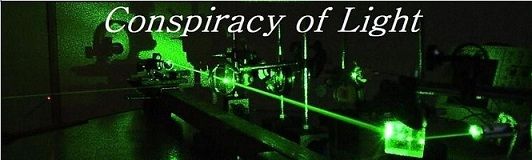The search for  new physics.

 A Single Laser One-Way Speed of light Experiment using a Standing Wave Interferometer Doug Marett (2011)  Introduction        A common theme often argued as contradicting the constancy of the speed of light postulate of relativity theory is the apparent anisotropy of light speed in rotating frames, such as in Sagnac interferometers. For an observer attached to the periphery of a rotating disk, beams of light counter-propagating around the rim of the disk will arrive back at the observer at different times. Such interferometers can detect the slow rotation of the earth at 15 degrees per hour, even though to the observer on the earth, the device appears completely stationary.  The phenomenon also manifests itself in the one way propagation times in GPS to earth-bound receivers, where the translational velocity of the earth at 30km/s is invisible, but the rotational velocity of the earth at 100x less becomes visible in the frame of the earth observer. This error time has become known as the Sagnac delay in GPS. This phenomenon has led some researchers to suspect that the Earth Centered Inertial (ECI) frame of the earth is isotropic for the speed of light, and the earth observers rotate through it. If this were the case, then one would expect the one way velocity of light would differ by c+v to the west of a rotating observer, and c-v to the east, where v is the rotational velocity of the earth at the observer’s latitude. Still other researchers have suggested that the velocity of the earth with respect to the Cosmic Microwave Background (CMB) should be detectable, since this is the only frame in which light is isotropic (a static Lorentz ether model).        However, attempting to measure the one-way translational speed of light with a conventional interferometer is considered impossible. Hendrik Lorentz demonstrated over 100 years ago that in Michelson-type interferometers any first order difference is the speed of light in different directions will perfectly cancel out at the photo-detector, and hypothesized that the Lorentz contraction cancels any second order differences.      Standing wave interferometers circumvent this dilemma. In a standing wave interferometer, one measures the position of the standing wave with respect to the plane of a transparent sensor. Differences in the speed of light in different directions in space should lead to the overall wavelength of the laser beam changing, since C=f l. If the speed of light rises or falls in a given direction, then the wavelength must also rise or fall (frequency can’t change since this would disrupt the continuity of the wave-train). This is detected as a phase difference between two counter-propagating waves, which is ultimately detected as a position change of a standing wave with respect to the standing wave detector (SWD). This can best be understood by the example below. What is shown is a Michelson interferometer combined with a standing wave sensor and dithered mirror. To visualize the difference between the two interferometers, imagine that a piece of glass is inserted into the path as shown below. Since the speed of light in the glass is lowered, the wavelength is also lowered. Since the beam goes through the SWD to a mirror and then returns, the standing wave formed  at the SWD will change its phase visibly as the wave crests recede through it due to the placement of the glass in the beam.  Since this standing wave is also being re-phased by the motion of the dithered mirror, this change in the phase relation between the two beams will manifest as a change in the phase position of the SWD signal output with respect to the dither signal driving the mirror, when they are displayed together on a 2-channel oscilloscope.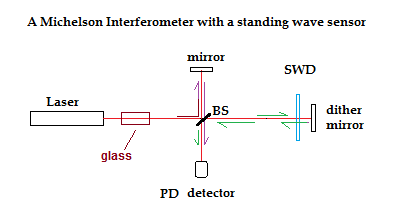Conversely, for the beams arriving at the photo-detector (PD) , by the time they reach it they are travelling in the same direction, and thus the phase change caused by the placement of the glass in the beam as shown will be equal for both beams returning from their respective arms and no net change in their phase relationship will be visible. Thus a wavelength change in the path common to both beams is visible in a standing wave interferometer but invisible in a Michelson interferometer. However, if the frequency of the laser beam changes throughout the entire system (and thereby the wavelength) then this kind of change can be detected even with a Michelson interferometer, as long as the two Michelson interferometer arms are of differing lengths, since this will generate a temporary phase shift between the beams arriving at PD. However, the fringe shift at SWD will be greater, since the phase differences due to the common paths will add when the beams countertravel through the SWD, rather than canceling out when they co-travel to the PD.     Since the standing wave difference results in a small D.C. voltage with respect to a much larger photovoltaic voltage from the beam in general, dithering one mirror in the optical path is the most common method of making this phase shift visible on an oscilloscope, since this will generate an oscillating AC signal as the standing wave dithers through the SWD detector.     A hypothetical aether wind would also cause a velocity change in the speed of light and thereby a wavelength change, and this should also be theoretically possible to visualize using a standing wave detector. In this paper we measure this changing phase relationship at the SWD while the entire optical table is rotated around 360 degrees. If the wavelengths of the counter-propagating beams change as predicted, then a phase displacement should similarly arise by rotating the table with respect to the direction of an aether wind. A small aether wind velocity of 336 m/s due to earth’s rotation will cause a fringe shift at the SWD of 7 full fringes after rotating the table 180 degrees, assuming that one path is longer in a single linear direction than the other by 1 meter.     This experiment became possible due to our obtaining a high-quality standing wave detector, graciously provided to us by the Institute of Photovoltaics, Jurlich. This sensor is a transparent NIP photodiode array of amorphous silicon with a total of 25 independent elements. It was designed for use at 632.8 nm, where reflections are minimized. The amorphous layer system thickness is less than 100 nm. A view of the Standing Wave Sensor (SWD) in its optical mount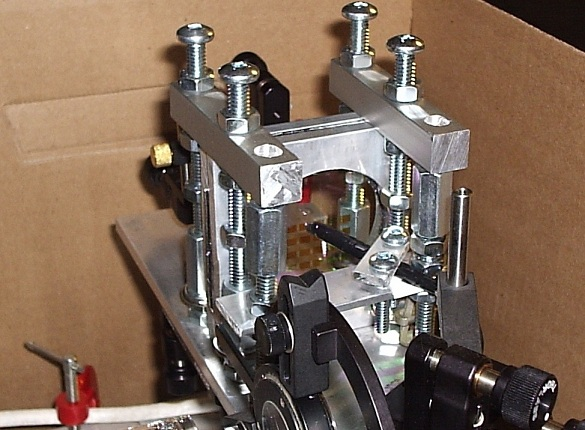Design of the Experiment    Four different interferometer arrangements were tested. A schematic representation of each is shown below. All of the interferometers used circularly polarized light and CPL filters to prevent light getting back into the laser cavity. Occasionally linearly polarized light was also tried, with similar results. In all cases there was one mirror which was typically dithered at 100 Hz. The location of this mirror varied from one arrangement to another. A frequency stabilized HeNe laser was used in all arrangements. This is critical, since each interferometer acts as a wavelength detector. The un-stabilized HeNe laser sweeps through its gain curve rapidly on first start-up, and then progressively more slowly after warming up for ½ hour or more.  When un-stabilized, the wavelength of the laser light is constantly changing, and thus the standing wave pattern at the SWD detector is constantly sweeping through fringes. This is used to advantage to calibrate the interferometers. Since we know the frequency sweep across the gain curve for the laser (640 MHz, based on direct measurements) we can calculate the wavelength change expected as the laser sweeps from one side of the gain curve to the other. This value can then be compared to the actual fringe shifts observed, and tested against an Excel spreadsheet model of the fringe shift expected based on the lengths of the combined optical paths. The laser can then be switched to lock mode, where the fringes stabilize in relation to the dither phase of the mirror oscillator.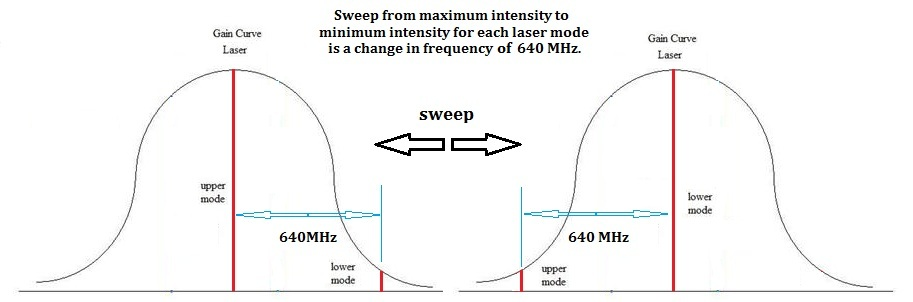In the first interferometer, the SWD output was sufficiently intense to see on the oscilloscope without amplification (around 20-50mV peak to peak). With the other interferometers, some amplification and filtering was necessary due to the weaker intensity of the output signal.  A 100Hz band-pass filter with a voltage gain of 100 was used for this purpose before the oscilloscope. Interferometer 1: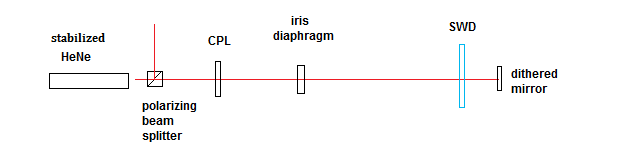This design used a conventional standing wave interferometer setup with a single linear path and a dithered mirror at the far end serving as the source of the return path through the SWD. This arrangement was found to generate dither signals from retro-reflections that did not include the path between the laser and the SWD detector – inserting a glass slide between the SWD detector and the dithered mirror generated a large fringe shift, but inserting the glass slide at other places in the beam had little to no effect. It also did not conform to the predictions from our excel spreadsheet as to the number of fringe shifts expected as the laser sweeps through its gain curve. As a result of these discrepancies, this design was abandoned.   Interferometer 2:In this second design, the return path through the SWD sensor was chosen to follow a different approach, using an independent arm to the dithered mirror.  This interferometer has one beam stationary through the detector, while the return beam oscillates back and forth with respect to it.  This design did conform to the excel spreadsheet predictions for the calibration fringe shift due to the laser frequency sweep, and all points in the path were sensitive to the glass slide test. Interferometer 3: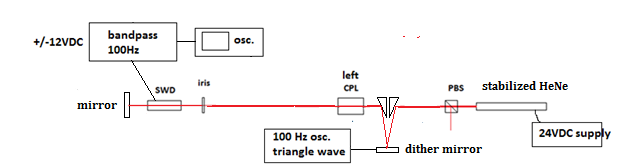In this third configuration, the dither mirror was moved to the laser side of the SWD detector. This configuration causes both beams (forward and return) to move in unison through the detector when the mirror is dithered. This was done to see if the result is any different from when only one beam moves. Again, this interferometer conformed to the predictions of our excel spreadsheet and was sensitive at all points to the glass slide test. Interferometer 4: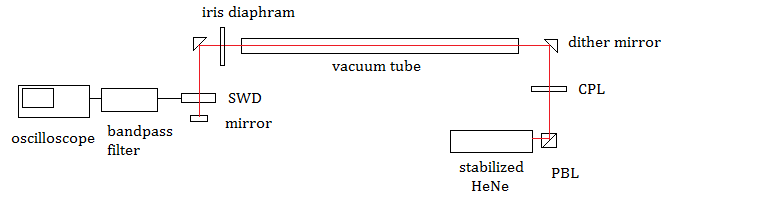Finally, in this last test, we added a vacuum path of 91 cm, to determine if the result of experiment 2 and 3 was affected by the presence of air, since air might be considered a moving dielectric medium for the light that drags the light at the rotational speed of the earth. A specially designed vacuum tube evacuated and sealed at 5X10-5 Torr was constructed for this purpose. Procedure     The general procedure on start-up was to turn on the laser and after a few minutes to count the number of fringe shifts that occur when the laser intensity sweeps from a minimum on the chosen mode to a maximum. This was observed using a multi-meter displaying the intensity of the waste beam polarized components with respect to one another. After at least ½ hour warm up, the laser would be put into lock mode. A two channel oscilloscope was used to display the triangle wave driving the dithered mirror and the SWD output signal (after amplification and filtering). An example of the two waveforms displayed on the oscilloscope is shown below:The intensity of the mirror driving voltage is always adjusted to the minimum required to display a dither signal of the same frequency as the triangle wave. Increasing the swing of the mirror leads to the dither signal becoming an integer multiple of the triangle wave driving signal, due to the mirror passing through multiple wavelengths per swing.     It was noted that depending on the optical adjusts of the interferometer, it was possible to separate two or more dither signals of different characteristics – most often there was one signal generated from the light passing down and back through the planed optical route, but sometimes another dither signal could be tuned in that appeared to be due to an internal reflection from one of the optical faces. In order to distinguish between these spurious signals and the desired one, it was necessary to put a glass slide between each of the optical components in sequence after changing an arrangement, to insure that each optical path segment was sensitive to a change in refractive index and thus would generate a fringe shift.  The optical paths were then adjusted to maximize this signal and detune any others. The resulting dither signal was generally incredibly sensitive to vibrations of any kind, as well as air currents around the apparatus. Typically a cardboard enclosure was used to prevent air currents from disturbing the interferometer, particularly during rotation.    Several methods of rotating the optical breadboard were tried. The first consisted of a swivel mount bearing mounted under a wooden slab – this bearing proved to be too noisy, and eventually a pedestal mount was used with the optical breadboard mounted directly on top. This was much smoother in rotation. In order to get a somewhat vibration free rotation of the breadboard through the cardinal directions, usually a chord was wrapped around the pedestal mounts sufficiently to generate a tension – the chord was connected to the platform on which the breadboard rested, and this tension was used to uniformly pull the device around as the chord unwound. Generally this procedure was repeated at least 50 times for each arrangement, with the total swing varying between 45 and 180 degrees. The fringe shift was noted over the course of each swing, as well as the degrees of the compass traversed. This data was then tabulated into a radar plot showing the average fringe shift, covering all 360 degrees.    Photographs of the four interferometer designs are shown below, the first two shown on the original swivel mount, while the second two are shown on the pedestal mount inside of the cardboard shielding used to block air currents for disrupting the interference pattern at the SWD.    Interferometer 1: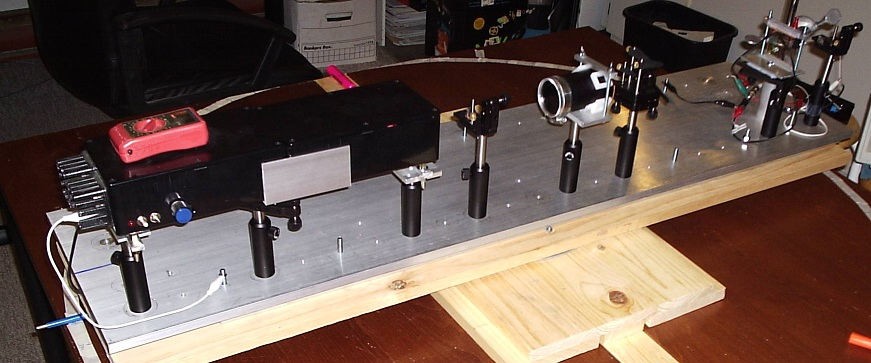Interferometer 2:Interferometer 3: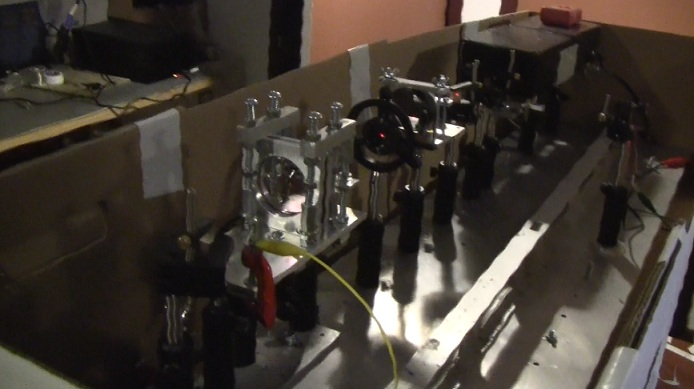Interferometer 4: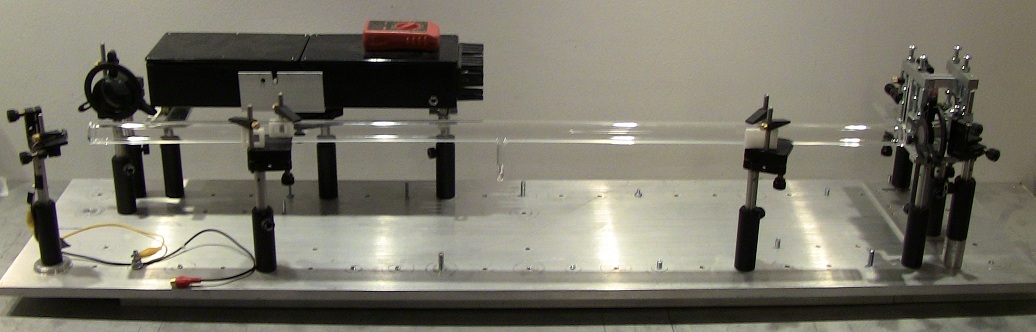The interferometers were also levelled around all 360 degrees to reduce the incidence of gravity related stresses on the optical components. Predicted Outcomes      Since these standing wave interferometers serve as very sensitive one-way wavelength of light detectors, what kind of output change would we expect if the one-way speed of light is different in various cardinal directions? Since C=f l, we would anticipate that the fringe shift around 360 degrees due to a wavelength change would correspond to the change in the one way velocity of light in the same directions. However, this fringe shift would not be linear, since the rate of change in the fringes with angle should depend on the Sine of the angle with the direction of the aether drift. In other words, although the wavelength of light would be shortest when the long axis of the interferometer is pointing into the oncoming aether drift (and longest when pointing in the opposite direction), the rate of change of the wavelength will be greatest when the long axis sweeps through the perpendicular line to the direction of aether motion. This is displayed in the diagram below. The blue line represents the rate of change of the fringe shift for a hypothetical aether wind coming from the east (9= 90 degrees on the compass). At the direction where the interferometer long axis crosses over the E or W line, the fringe shift slows down, halts, and then reverses direction, following the change in the detected wavelength.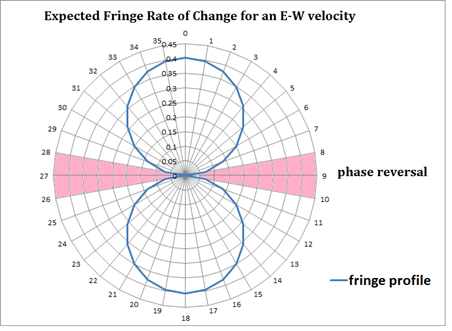The diagram above is thus what we would expect if we were detecting the rotational motion of the interferometer with respect to a stationary aether fixed to the ECI frame of the earth. We would also expect such a pattern if the interferometer was swept through a aether drift due to our motion with respect to the Cosmic Microwave Background (CMB) except that the phase reversal point would correspond to the direction towards the constellation Leo and would vary with direction consistent with the change in the sidereal space direction with respect to our compass. Also, the fringe shift due to our motion with respect to the CMB would be at a maximum approximately 2000x larger than that expected for the rotational velocity of the earth, if it is detectable. A third possibility is that the wavelength of light is isotropic with respect to the rotating earth (i.e. the ECEF frame) at a given latitude, a result which would support a Stokes aether model, or relativity, or any model of light where the one-way change in the wavelength of light due to motion is undetectable. Experimental Results:     The experimental results for interferometer 2 are shown below. The low fringe change around 360 degrees of rotation immediately ruled out any detectable wavelength change due to our motion with respect to the CMB or our orbital motion.  The expected rate of change of the fringe pattern for an E-W velocity of 336 m/s is shown below in blue. The expected fringe shift around 360 degrees was 11.9 full fringes. The actual fringe shift was 4.94 (red) and appeared to be random and due to the vibrational motion of the interferometer as it turned on its platform. No distinct phase reversals or rate of change pattern was observed. This suggests that no aether wind was detected in any direction with a velocity greater than our noise level of 139 m/s.  n = 25.The experimental results for interferometer 3 are somewhat similar.  For 83 determinations, the average was a uniform fringe shift of 2.79 over 360 degrees, whereas we expected 9.2 full fringes for a velocity of 336 m/s due to the rotating earth at our latitude. This puts the maximum velocity possible at less than our noise level of 102 m/s.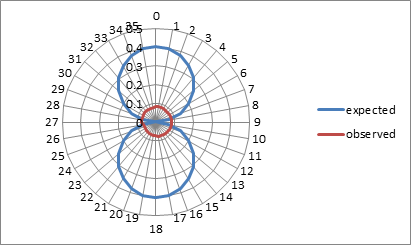Since the results were considerably smaller even than would be expected for the rotational velocity of the earth at 336 m/s at our latitude, it was decided to test to see if the atmosphere of the earth itself might be dragging the light, much like other dielectric mediums drag light  (fibre optic cable, water, etc.), the experiment of Fizeau being a notable example. This might be unlikely, since the Fresnel drag equation does not suggest there would be substantial drag for air with a refractive index of very close to 1, but we decided to rule it out as a possibility nonetheless. Assuming full drag by air, and no drag by a vacuum, we would expect a fringe shift of 12.88 over 360 degrees of rotation, based on our vacuum path of 91 cm in length. The results of these tests are shown below, n = 50. The actual fringe shift was much lower again, at 2.97 fringe shifts, corresponding to a maximum velocity of not more than 77 m/s.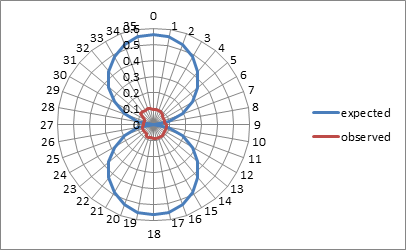Discussion      The experimental results do not conform to our original expectation that an aether wind generated fringe shift would be visible. We found that the one way speed of light appears to be isotropic, at least down to our noise threshold of 77-139 m/s, depending on the arrangement. Further, with interferometer 3 we did observe at least once a fringe shift of zero, for each chosen direction of the compass. . The apparent “null” result however, after careful analysis, can be found to be explained entirely by the expectations of Lorentz ether theory (LET).   A frequency shift on the laser would be expected due to its rotational movement around the platform axis with respect to any hypothetical aether wind. This is because a moving clock experiences a time dilation effect – Lorentz ether theory sees this as a clock error caused by the motion of the clock with respect to a preferred frame for light, whereas relativity interprets this time dilation as a real change in the passage of time for the clock. This frequency shift should be equal and opposite the fringe shift along the optical path using Lorentz ether theory. This was the criticism put forward by Tyapkin to explain why the Cialdea experiment, and virtually all one way speed of light tests cannot generate a fringe shift as might be anticipated.  This was also pointed out by Ruderfer in explaining the lack of a fringe shift in Mossbauer tests. The method of Tyapkin, as applied to this particular experiment, predicts that the laser will experience a time dilation of: Dt = t*(1/(1-v2/c2)*(L/2c2)*V*wrot*sin(q)   Resulting in an ultimate counteracting fringe shift along the optical path of: Dfringe = t*(1/(1-v2/c2)*(L/2cl)*V*wrot*sin(q) Where: t = rotation time (s)                    wrot = angular velocity of the laser v = aether wind (m/s),                sin(q) = average sine value over 90 degrees x 2 = -0.636619783 x 2 c = speed of light (m/s),              L = one way optical path length (m) The fringe shift would then be double this amount since in the standing wave interferometer the fringe shift from the return beam adds to the amount of the forward beam rather than cancelling. This equation was put into a simulator of the interferometers used in this experiment. One example is interferometer 3; the simulator can be found here. As can be seen from this simulator, the fringe shift along the optical path due to the rotation of the interferometer with respect to an aether wind is perfectly cancelled by the frequency change of the laser due to the time dilation involved in its motion. This is regardless of the angular velocity of the laser or its position on the optical table with respect to the axis.      Interestingly, by this same argument, the relativistic interpretation of this experiment would actually predict a fringe shift. Using relativity, there would be no fringe shift along the path since the speed of light would be predicted to be c in all directions. However, the laser frequency shift caused by the rotational motion of the interferometer would cause a fringe shift proportional to v2/2c2, where v is the tangential velocity of the laser. This should be significant enough to measure in some high rotational speed experiments.     It should be noted that a similar argument can be made to explain the null result of one-way velocity of light experiments involving two lasers. A spreadsheet simulator of this kind of experiment is given here. Given the ultimate resolution of this experiment at around 1 fringe shift over 180 degrees rotation, there is not sufficient resolution to distinguish between the null result predicted by Lorentz Ether theory or the very small positive result predicted by relativity. Conclusions      In the original premise of this experiment in was believed that this interferometer would be successful in detecting an aether drift if a preferred medium of space exists. However, , as has been explained in the discussion, this anticipated result turns into a null result when one takes into account the frequency shift of the laser clock due to its rotational motion around the table. This frequency shift is called for in both Lorentz ether theory and relativity, and thus within the resolving power of this experiment we cannot distinguish between the predicted result of the two theories. This same frequency shift in the rotating clock is the reason why other one-way speed of light experiments with rotating lasers, masers, or using the Mossbauer effect cannot distinguish between the two theories. References [.1.]    A. A. Tyapkin, Lett. Nuovo Cimento, 7, 15, pp. 760-4, (1973).   [.2.]    H. Lorentz, Lectures on Theoretical Physics, V.1 pp. 14-19 (1927).   [ 3 ]    J. Larmor, Aether and Matter, Cambridge University Press (1900).   [ 4 ]    A. Michelson, E. Morley, Am. J. Sci, 34, 203, pp. 333–345 (1887).   [ 5 ]    R. Mansouri, R. Sexl, Gen. Rel. Grav., 8, 10, pp. 809-14 (1977).   [ 6 ]    C. Moller, Suppl. Nuovo Cimento, 6, pp. 381 (1957).   [ 7 ]    M. Ruderfer, Phys. Rev. Lett. , 5, 5, pp.191-2 (1960).   [ 8 ]    K. Turner, H. Hill, Bull Am. Phys. Soc., 8, pp. 28 (1963).   [ 9 ]    D. Champeney, et. al., Phys. Lett., 7,4 ,pp.241-3 (1963).       E. Preikschat, The Mossbauer Effect and Tests of Relativity,            Thesis, U. of Birmingham, 1968, Chapter 4-10.       R.  Cialdea, Lettere Al Nuovo Cimento, 4, 16, pp.821 (1972).       R. Hatch, Proc. 58th Ann. Meeting Inst. Nav. pp. 70-81. (2002).       M. Ruderfer, Phys. Rev. Lett. , 7, 9, pp. 361 (1961).       R. Mansouri, R. Sexl, Gen. Rel. Grav., 8, 7, pp. 497-513 (1976).       D. Marett, A Replication of the Cialdea One-Way Speed of Light            Experiment (2010).  http://www.conspiracyoflight.com/Cialdea/Cialdea.html       E.W. Silvertooth, Spec. Sci. Tech. Vol.10, No.1, pp.3-7.